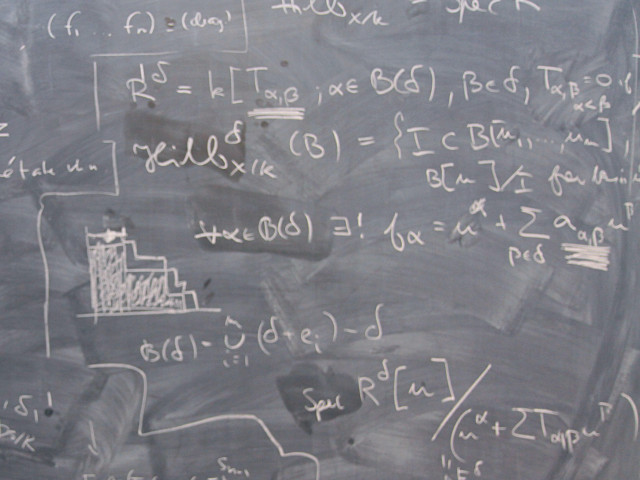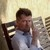# SF1604 Linear Algebra 7.5 creditsCourse of basic linear algebra and some elementary algebraic concepts.

Course offering missing for current semester as well as for previous and coming semesters
Headings with content from the Course syllabus SF1604 (Autumn 2008–) are denoted with an asterisk ( )

## Content and learning outcomes

### Course contents

Complex numbers, polynomials, proof by induction. Systems of linear equations, real and complex matrices and determinants; Cramer's rule. Adjoint and inverse matrices. Vector geometry in R2 and R3, cross product and dot product. Generalizations to higher dimensions. Gram-Schmidt orthogonalization and projections in Rn. General vector spaces and inner product spaces. Linear transformations between vector spaces, eigenvalues and eigenvectors, quadratic forms. Change of basis and matrix representation of linear transformations and quadratic forms in different bases. Diagonalization of matrices, the spectral theorem for symmetric matrices.

### Intended learning outcomes

The general objective is to give basic knowledge in linear algebra and such elementary algebra as complex numbers, polynomial equations and proofs by induction. More precisely after a course is expected that the students:

• know how to solve linear equations by using Gauss elimination.
• know how to perform matrix calculations.
• know how to calculate the determinant of a matrix both by elementary row and column operations as well as by expanding by a row or a column.
• understand the concept of vector and how to add a vectors and multiply them with scalar in a space of dimension 3, both by using coordinates and in spaces without a given coordinate system.
• know the formula for the dot product of vectors, both using a given orthonormal coordinate system and without any coordinate system.

Further

• be able to use the properties for the dot product.
• be a master of the cross product.
• be able to calculate distances, angles between vectors, the area and the volume of simple geometric objects limited by planes and lines.
• be a master of the parametric form of a line and the equation of a plane, and by using these concepts be able to solve simple geometrical problems concerning planes and lines as e.g. the distance between lines, the intersection point between a line and a plane, the distance between a point and a plane, the projection of vectors on lines and planes.
• understand the concept linear vector space and concepts like subspace, linear span, linearly independent, set of base vectors, dimension and coordinates.
• know how to calculate the rank of a matrix and be a master of the connection between the rank and the dimension of the null space of a matrix.
• be a master of the solvability of system of linear equations and the existence of the inverse of a matrix by using the concepts rank of a matrix and the value of the determinant of a matrix.
• know the definition of an inner product space and be a master of the problem
• to decide if a product is an inner product
• by using the Gram Schmidt method be able to find an orthogonal base in a subspace of an inner product space, and to be able to characterize orthogonal matrices.
• be a master of the use of the least square method to find optimal solutions of inconsistent linear equations.
• be a master of the use of bases of changes matrices for the description of the change of base in a vector space.
• know how to find eigenvalues and eigenvectors and eigenspaces of a matrices, and be able to perform such calculations for small matrices and know how to diagonalize a matrix.
• be a master of the spectral theorem and some consequences of that theorem.
• be able to decide whether or not a map between vector spaces is a linear map and be a master of the determination of the matrix of a linear map.
• be able to, by using the method with eigenvalues, to decide whether or not a quadratic form is positive definite and further be able to characterize quadric surfaces.
• be a master of complex numbers, including the addition, the subtracting, the multiplication and the division, the modulus, polar form and how the multiplication and division can be performed in polar form.
• be a master of the use of DeMoivre's theorem and the solution of binomial equations
• be a master of the use of the mathematical induction to solve simple mathematical problems devoted for induction.
• be a master of the fundamental theorem of algebra, especially the connection between zeros and factors of polynomials, especially for real polynomials.

Finally, as a consequence of the mathematics that have been studied during the course, the general skill for mathematics is expected to have been improved, especially for the purpose of the understanding how a theory of mathematics can be developed from axioms, definitions, theorems and proofs. More particularly it is expected that the skill for understanding terminology and arguments, connected to linear algebra and occurring in other courses as well as in other scientific contexts, has been improved.

### Course disposition

No information inserted

## Literature and preparations

### Specific prerequisites

Advanced mathematics (NT from high school level).

### Recommended prerequisites

No information inserted

### Equipment

No information inserted

### Literature

No information inserted

## Examination and completion

If the course is discontinued, students may request to be examined during the following two academic years.

A, B, C, D, E, FX, F

### Examination

• TEN1 - Examination, 7.5 credits, grading scale: A, B, C, D, E, FX, F

Based on recommendation from KTH’s coordinator for disabilities, the examiner will decide how to adapt an examination for students with documented disability.

The examiner may apply another examination format when re-examining individual students.

### Other requirements for final grade

Written exam (TEN1; 7,5 hp).

### Opportunity to complete the requirements via supplementary examination

No information inserted

### Opportunity to raise an approved grade via renewed examination

No information inserted

### ExaminerRoy Skjelnes

### Ethical approach

• All members of a group are responsible for the group's work.
• In any assessment, every student shall honestly disclose any help received and sources used.
• In an oral assessment, every student shall be able to present and answer questions about the entire assignment and solution.

## Further information

### Course web

Further information about the course can be found on the Course web at the link below. Information on the Course web will later be moved to this site.

Course web SF1604

SCI/Mathematics

### Main field of study

Mathematics, Technology

First cycle